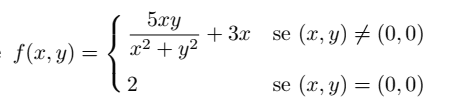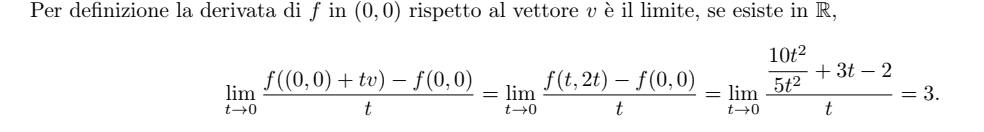# Problem with a directional derivative calculation

Amaelle
Homework Statement:
the directional derivative of the function f(x,y) in respect to the point(0,0) according to the vector v(1,2) is equal to
Relevant Equations:
directional derivative
this is the functionand this is the solution in which the definition has been usedmy question is
Why we can not use the traditional approach? I mean calculation the partial derivative which equals 0 in our case? And doing the dot product with the vector v (after normalizing it)

•Delta2

Homework Helper
Gold Member
The traditional approach ( i suppose you mean to calculate ##\nabla f (0,0)\cdot \vec{v}## )seems more complex to me.

But i am afraid there is another problem here too: The traditional approach can be used only when the function is differentiable at the point of interest, but i am afraid this function isn't differentiable at (0,0) (from a quick look I see that even the partial derivatives at (0,0) don't exist).

So if indeed the partial derivatives don't exist at (0,0) then we just can't use the traditional approach anyway. I hope I didn't blunder on this, can you show me how you calculate the partial derivatives at (0,0) cause I get infinities...

Last edited:
••Amaelle and fresh_42
Homework Helper
Gold Member
2022 Award
The function is not even continuous at ##(0,0)##.

•Amaelle and Delta2
Amaelle

Homework Helper
Gold Member
2022 Award
The answer given still makes no sense. It ignores the 2/t term in taking the limit.

Homework Helper
Gold Member
It ignores the 2/t term in taking the limit
I see two 2/t term, one positive and one negative and they cancel each other.

Homework Helper
Gold Member
2022 Award
I see two 2/t term, one positive and one negative and they cancel each other.
Ah, yes, ok.
But the answer is still wrong. t is an arbitrary parameter. It makes no sense to divide by t; need to consider the length in the given direction.
Given that we know the limit of the function is zero, making it continuous, in the given direction, it is simpler to switch to polar:
##f=5\sin(\theta)\cos(\theta)+3r\cos(\theta)##
Diff wrt r and plug in the value of theta.

Homework Helper
Gold Member
Ah, yes, ok.
But the answer is still wrong. t is an arbitrary parameter. It makes no sense to divide by t; need to consider the length in the given direction.
That's the definition of the directional derivative, one divides by ##t## there. By the way I see it ,##t## is the length in the direction of ##\vec{v}##.

In the normalized version you divide also by ##|\vec{v}|##.

Homework Helper
Gold Member
2022 Award
That's the definition of the directional derivative
So the answer depends on the magnitude of ##\vec v##? Strange, but I stand corrected.

Homework Helper
Gold Member
So the answer depends on the magnitude of ##\vec v##? Strange, but I stand corrected.
Well yes i guess the non normalized version depends on the magnitude of the vector, though i can't imagine how we can prove that the dependency is always linear.

There are two different conventions, one that ##\nabla_{\vec{v}} f(\vec{x}) = \nabla f(\vec{x}) \cdot \vec{v}##, and the other that ##\nabla_{\vec{v}} f(\vec{x}) = \nabla f(\vec{x}) \cdot \left(\vec{v}/|\vec{v}| \right)##. I was taught that the first convention is more common (##\nabla_{\vec{v}} f(\vec{x}) = \nabla f(\vec{x}) \cdot \vec{v}##), but that you should be aware of the other convention.

•Delta2 and PeroK
Homework Helper
Gold Member
There are two different conventions, one that ##\nabla_{\vec{v}} f(\vec{x}) = \nabla f(\vec{x}) \cdot \vec{v}##, and the other that ##\nabla_{\vec{v}} f(\vec{x}) = \nabla f(\vec{x}) \cdot \left(\vec{v}/|\vec{v}| \right)##. I was taught that the first convention is more common (##\nabla_{\vec{v}} f(\vec{x}) = \nabla f(\vec{x}) \cdot \vec{v}##), but that you should be aware of the other convention.
According to wikipedia (and i think also according to my vector calculus notes of 199x) neither of the above is the definition of the directional derivative. The above we can prove from the definition, if the function f is differentiable.

•Amaelle and etotheipi
According to wikipedia (and i think also according to my vector calculus notes of 199x) neither of the above is the definition of the directional derivative. The above we can prove from the definition, if the function f is differentiable.

In Physics, everything is differentiable••archaic and Delta2
Homework Helper
Gold Member
In Physics, everything is differentiableWell yes i know what you saying here , but it is just that in physics we consider a function differentiable when all the partial/total derivatives exist. In math and with functions of many variables, differentiability is a stronger condition than that
https://en.wikipedia.org/wiki/Differentiable_function#Differentiability_in_higher_dimensions

••etotheipi and Amaelle